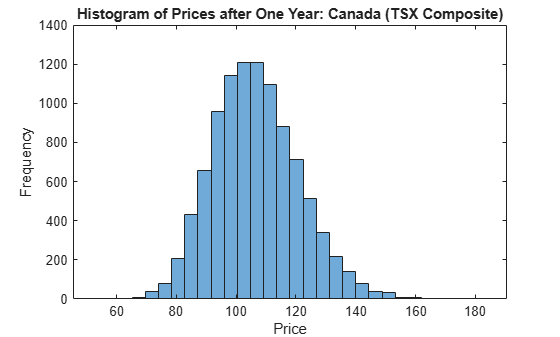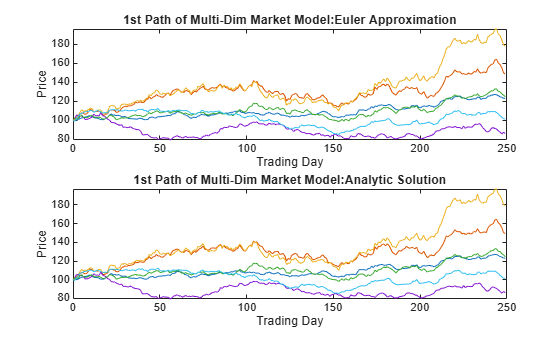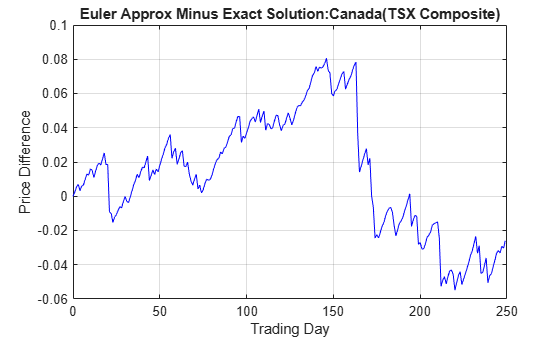simBySolution

Simulate approximate solution of diagonal-drift GBM processes

Description

example

[Paths,Times,Z] = simBySolution(MDL,NPeriods) simulates approximate solution of diagonal-drift for geometric Brownian motion (GBM) processes.

example

[Paths,Times,Z] = simBySolution(___,Name,Value) adds optional name-value pair arguments.

Examples

collapse all

Use GBM simulation functions. Separable GBM models have two specific simulation functions:

• An overloaded Euler simulation function (simulate), designed for optimal performance.

• A simBySolution function that provides an approximate solution of the underlying stochastic differential equation, designed for accuracy.

Load the Data_GlobalIdx2 data set and specify the SDE model as in Representing Market Models Using SDE Objects, and the GBM model as in Representing Market Models Using SDELD, CEV, and GBM Objects.

prices  = [Dataset.TSX Dataset.CAC Dataset.DAX ...
Dataset.NIK Dataset.FTSE Dataset.SP];

returns =  tick2ret(prices);

nVariables  = size(returns,2);
expReturn   = mean(returns);
sigma       = std(returns);
correlation = corrcoef(returns);
t           = 0;
X           = 100;
X           = X(ones(nVariables,1));

F = @(t,X) diag(expReturn)* X;
G = @(t,X) diag(X) * diag(sigma);

SDE = sde(F, G, 'Correlation', ...
correlation, 'StartState', X);

GBM = gbm(diag(expReturn),diag(sigma), 'Correlation', ...
correlation, 'StartState', X);

To illustrate the performance benefit of the overloaded Euler approximation function (simulate), increase the number of trials to 10000.

nPeriods = 249;      % # of simulated observations
dt       =   1;      % time increment = 1 day
rng(142857,'twister')
[X,T] = simulate(GBM, nPeriods, 'DeltaTime', dt, ...
'nTrials', 10000);

whos X
Name        Size                     Bytes  Class     Attributes

X         250x6x10000            120000000  double

Using this sample size, examine the terminal distribution of Canada's TSX Composite to verify qualitatively the lognormal character of the data.

histogram(squeeze(X(end,1,:)), 30), xlabel('Price'), ylabel('Frequency')
title('Histogram of Prices after One Year: Canada (TSX Composite)')Simulate 10 trials of the solution and plot the first trial:

rng('default')
[S,T] = simulate(SDE, nPeriods, 'DeltaTime', dt, 'nTrials', 10);
rng('default')
[X,T] = simBySolution(GBM, nPeriods,...
'DeltaTime', dt, 'nTrials', 10);
subplot(2,1,1)
title('1st Path of Multi-Dim Market Model:Euler Approximation')
subplot(2,1,2)
title('1st Path of Multi-Dim Market Model:Analytic Solution')In this example, all parameters are constants, and simBySolution does indeed sample the exact solution. The details of a single index for any given trial show that the price paths of the Euler approximation and the exact solution are close, but not identical.

The following plot illustrates the difference between the two functions:

subplot(1,1,1)
plot(T, S(:,1,1) - X(:,1,1), 'blue'), grid('on')
title('Euler Approx Minus Exact Solution:Canada(TSX Composite)')The simByEuler Euler approximation literally evaluates the stochastic differential equation directly from the equation of motion, for some suitable value of the dt time increment. This simple approximation suffers from discretization error. This error can be attributed to the discrepancy between the choice of the dt time increment and what in theory is a continuous-time parameter.

The discrete-time approximation improves as DeltaTime approaches zero. The Euler function is often the least accurate and most general method available. All models shipped in the simulation suite have the simByEuler function.

In contrast, the simBySolution function provides a more accurate description of the underlying model. This function simulates the price paths by an approximation of the closed-form solution of separable models. Specifically, it applies a Euler approach to a transformed process, which in general is not the exact solution to this GBM model. This is because the probability distributions of the simulated and true state vectors are identical only for piecewise constant parameters.

When all model parameters are piecewise constant over each observation period, the simulated process is exact for the observation times at which the state vector is sampled. Since all parameters are constants in this example, simBySolution does indeed sample the exact solution.

For an example of how to use simBySolution to optimize the accuracy of solutions, see Optimizing Accuracy: About Solution Precision and Error.

Input Arguments

collapse all

Geometric Brownian motion (GBM) model, specified as a gbm object that is created using gbm.

Data Types: object

Number of simulation periods, specified as a positive scalar integer. The value of NPeriods determines the number of rows of the simulated output series.

Data Types: double

Name-Value Arguments

Specify optional comma-separated pairs of Name,Value arguments. Name is the argument name and Value is the corresponding value. Name must appear inside quotes. You can specify several name and value pair arguments in any order as Name1,Value1,...,NameN,ValueN.

Example: [Paths,Times,Z] = simBySolution(GBM,NPeriods,'DeltaTime',dt,'NTrials',10)

Simulated trials (sample paths) of NPERIODS observations each, specified as the comma-separated pair consisting of 'NTrials' and a positive scalar integer.

Data Types: double

Positive time increments between observations, specified as the comma-separated pair consisting of 'DeltaTimes' and a scalar or a NPERIODS-by-1 column vector.

DeltaTime represents the familiar dt found in stochastic differential equations, and determines the times at which the simulated paths of the output state variables are reported.

Data Types: double

Number of intermediate time steps within each time increment dt (specified as DeltaTime), specified as the comma-separated pair consisting of 'NSteps' and a positive scalar integer.

The simBySolution function partitions each time increment dt into NSteps subintervals of length dt/NSteps, and refines the simulation by evaluating the simulated state vector at NSteps − 1 intermediate points. Although simBySolution does not report the output state vector at these intermediate points, the refinement improves accuracy by allowing the simulation to more closely approximate the underlying continuous-time process.

Data Types: double

Flag to indicate whether simBySolution uses antithetic sampling to generate the Gaussian random variates that drive the Brownian motion vector (Wiener processes), specified as the comma-separated pair consisting of 'Antithetic' and a scalar logical flag with a value of True or False.

When you specify True, simBySolution performs sampling such that all primary and antithetic paths are simulated and stored in successive matching pairs:

• Odd trials (1,3,5,...) correspond to the primary Gaussian paths.

• Even trials (2,4,6,...) are the matching antithetic paths of each pair derived by negating the Gaussian draws of the corresponding primary (odd) trial.

Note

If you specify an input noise process (see Z), simBySolution ignores the value of Antithetic.

Data Types: logical

Direct specification of the dependent random noise process used to generate the Brownian motion vector (Wiener process) that drives the simulation, specified as the comma-separated pair consisting of 'Z' and a function or as an (NPERIODS * NSTEPS)-by-NBROWNS-by-NTRIALS three-dimensional array of dependent random variates.

The input argument Z allows you to directly specify the noise generation process. This process takes precedence over the Correlation parameter of the input gbm object and the value of the Antithetic input flag.

Note

If you specify Z as a function, it must return an NBROWNS-by-1 column vector, and you must call it with two inputs:

• A real-valued scalar observation time t.

• An NVARS-by-1 state vector Xt.

Data Types: double | function

Flag that indicates how the output array Paths is stored and returned, specified as the comma-separated pair consisting of 'StorePaths' and a scalar logical flag with a value of True or False.

If StorePaths is True (the default value) or is unspecified, simBySolution returns Paths as a three-dimensional time series array.

If StorePaths is False (logical 0), simBySolution returns the Paths output array as an empty matrix.

Data Types: logical

Sequence of end-of-period processes or state vector adjustments of the form, specified as the comma-separated pair consisting of 'Processes' and a function or cell array of functions of the form

${X}_{t}=P\left(t,{X}_{t}\right)$

The simBySolution function runs processing functions at each interpolation time. They must accept the current interpolation time t, and the current state vector Xt, and return a state vector that may be an adjustment to the input state.

simBySolution applies processing functions at the end of each observation period. These functions must accept the current observation time t and the current state vector Xt, and return a state vector that may be an adjustment to the input state.

The end-of-period Processes argument allows you to terminate a given trial early. At the end of each time step, simBySolution tests the state vector Xt for an all-NaN condition. Thus, to signal an early termination of a given trial, all elements of the state vector Xt must be NaN. This test enables a user-defined Processes function to signal early termination of a trial, and offers significant performance benefits in some situations (for example, pricing down-and-out barrier options).

If you specify more than one processing function, simBySolution invokes the functions in the order in which they appear in the cell array. You can use this argument to specify boundary conditions, prevent negative prices, accumulate statistics, plot graphs, and more.

Data Types: cell | function

Output Arguments

collapse all

Simulated paths of correlated state variables, returned as a (NPERIODS + 1)-by-NVARS-by-NTRIALS three-dimensional time series array.

For a given trial, each row of Paths is the transpose of the state vector Xt at time t. When the input flag StorePaths = False, simBySolution returns Paths as an empty matrix.

Observation times associated with the simulated paths, returned as a (NPERIODS + 1)-by-1 column vector. Each element of Times is associated with the corresponding row of Paths.

Dependent random variates used to generate the Brownian motion vector (Wiener processes) that drive the simulation, returned as a (NPERIODS * NSTEPS)-by-NBROWNS-by-NTRIALS three-dimensional time series array.

collapse all

Antithetic Sampling

Simulation methods allow you to specify a popular variance reduction technique called antithetic sampling.

This technique attempts to replace one sequence of random observations with another of the same expected value, but smaller variance. In a typical Monte Carlo simulation, each sample path is independent and represents an independent trial. However, antithetic sampling generates sample paths in pairs. The first path of the pair is referred to as the primary path, and the second as the antithetic path. Any given pair is independent of any other pair, but the two paths within each pair are highly correlated. Antithetic sampling literature often recommends averaging the discounted payoffs of each pair, effectively halving the number of Monte Carlo trials.

This technique attempts to reduce variance by inducing negative dependence between paired input samples, ideally resulting in negative dependence between paired output samples. The greater the extent of negative dependence, the more effective antithetic sampling is.

Algorithms

The simBySolution function simulates NTRIALS sample paths of NVARS correlated state variables, driven by NBROWNS Brownian motion sources of risk over NPERIODS consecutive observation periods, approximating continuous-time GBM short-rate models by an approximation of the closed-form solution.

Consider a separable, vector-valued GBM model of the form:

$d{X}_{t}=\mu \left(t\right){X}_{t}dt+D\left(t,{X}_{t}\right)V\left(t\right)d{W}_{t}$

where:

• Xt is an NVARS-by-1 state vector of process variables.

• μ is an NVARS-by-NVARS generalized expected instantaneous rate of return matrix.

• V is an NVARS-by-NBROWNS instantaneous volatility rate matrix.

• dWt is an NBROWNS-by-1 Brownian motion vector.

The simBySolution function simulates the state vector Xt using an approximation of the closed-form solution of diagonal-drift models.

When evaluating the expressions, simBySolution assumes that all model parameters are piecewise-constant over each simulation period.

In general, this is not the exact solution to the models, because the probability distributions of the simulated and true state vectors are identical only for piecewise-constant parameters.

When parameters are piecewise-constant over each observation period, the simulated process is exact for the observation times at which Xt is sampled.

Gaussian diffusion models, such as hwv, allow negative states. By default, simBySolution does nothing to prevent negative states, nor does it guarantee that the model be strictly mean-reverting. Thus, the model may exhibit erratic or explosive growth.

 Aït-Sahalia, Yacine. “Testing Continuous-Time Models of the Spot Interest Rate.” Review of Financial Studies 9, no. 2 ( Apr. 1996): 385–426.

 Aït-Sahalia, Yacine. “Transition Densities for Interest Rate and Other Nonlinear Diffusions.” The Journal of Finance 54, no. 4 (Aug. 1999): 1361–95.

 Glasserman, Paul. Monte Carlo Methods in Financial Engineering. New York: Springer-Verlag, 2004.

 Hull, John C. Options, Futures and Other Derivatives. 7th ed, Prentice Hall, 2009.

 Johnson, Norman Lloyd, Samuel Kotz, and Narayanaswamy Balakrishnan. Continuous Univariate Distributions. 2nd ed. Wiley Series in Probability and Mathematical Statistics. New York: Wiley, 1995.

 Shreve, Steven E. Stochastic Calculus for Finance. New York: Springer-Verlag, 2004.